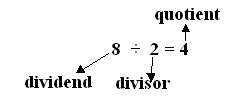Email us to get an instant 20% discount on highly effective K-12 Math & English kwizNET Programs!

#### Online Quiz (WorksheetABCD)

Questions Per Quiz = 2 4 6 8 10

### Grade 5 - Mathematics3.2 Divisor, Dividend, Quotient, and Remainder

 Multiplication: In multiplication two numbers are given and we find their product as 5 � 15 = ? 5 � 15 = 75 Division: We can rewrite the above multiplication as a division problem as: What number times 15 equals 75? ? � 15 = 75 This is a division problem. Division is also called the inverse of multiplication. To find the answer we have to divide as: ? = 75 � 15 Here we have to divide 75 into groups of 15 75 � 15 = 5 75 divided by 15 equals 5 Else we could subtract 15 from 75 five times. Multiplication is repeated addition and division is repeated subtraction. 15 is called the divisor. 75 is called the dividend. It is the number being divided. 4 is called the quotient. In a division problem: The number that is divided is called the dividend. The number that divides the dividend is called divisor. The number of times the dividend is divisible by the divisor is called the quotient. If the dividend is completely not divisible by the divisor it leaves behind a remainder. Example: How do you check if the division is right?Dividend = Quotient x Divisor + Remainder Here the remainder is 0 Hence 8 = 4 x 2 Answer: Multiply the quotient and the divisor Directions: Answer the following questions. Also write at least 5 examples of your own for division with remainder and division without remainder.
 Q 1: The number that is remaining after division problem is calleddivisorquotientdividendremainder Q 2: In the problem 30/5 = 6, what is the quotient?5630 Q 3: In the problem 30/5 = 6, what is the divisor?6305 Q 4: The remainder is less than the divisoralways falsesometimes falsesometimes truealways true Q 5: The answer to a division problem is the quotientfalsetrue Q 6: The number you are dividing is calleddivisordividendremainderquotient Q 7: In the problem 30/5 = 6, what is the dividend?3056 Q 8: If a division problem has no remainder,dividend = quotient*divisordivisor = dividend*quotientquotient = dividend*divisor Question 9: This question is available to subscribers only! Question 10: This question is available to subscribers only!

#### Subscription to kwizNET Learning System offers the following benefits:

• Unrestricted access to grade appropriate lessons, quizzes, & printable worksheets
• Instant scoring of online quizzes
• Progress tracking and award certificates to keep your student motivated
• Unlimited practice with auto-generated 'WIZ MATH' quizzes